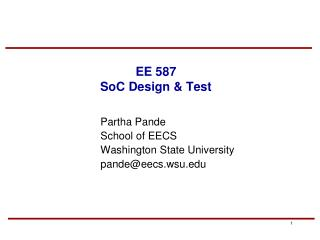DownloadDownload PresentationEE 587 SoC Design & Test

# EE 587 SoC Design & Test

Download Presentation## EE 587 SoC Design & Test

- - - - - - - - - - - - - - - - - - - - - - - - - - - E N D - - - - - - - - - - - - - - - - - - - - - - - - - - -
##### Presentation Transcript

1. EE 587 SoC Design & Test Partha Pande School of EECS Washington State University pande@eecs.wsu.edu

2. Midterm Review

3. Wire Characteristics • Wire modeling in details • Wire delay and buffer insertion • Uniform and non uniform buffer insertion • How scaling changes characteristics of a chip

4. Interconnect Capacitance Profiles • Total capacitance can be decomposed into three components: • Area capacitance • Lateral capacitance • Fringe capacitance V e r t i c a l s p a c i n g b e t w e e n H c o n d u c t o r s T S H e i g h t a b o e v S u b s t r a t e Horizontal spacing between conductors W H Ctotal = Carea + Clateral + Cfringe

5. Wire Dimensions • T=wire thickness, H=vertical wire separation, S=horizontal wire separation, W=wire width, L=wire length • T and H are fixed parameters based on the fabrication process • W, S and L are under the designer’s control

6. Computation of Area Capacitances • Area capacitance per unit length can be simply calculated using: Metal 2 Ca H W Metal 1 Ca Ca= ox W = 0.035fF/um (W/H) H

7. Computation of Lateral Capacitances • Lateral capacitance per unit length for closely spaced wires can be calculated using: • For widely spaced wires, CL drops off as 1/S Metal 2 Closely spaced wires S T Metal 1 CL CL CL= ox T = 0.035fF/um (T/S) s

8. Computation of Fringe Capacitances Metal 2 Ca Widely separated wires H Cf Cf T Metal 1 Ca For widely spaced conductors

9. Wire Inductance • Wide wires in clock distribution & upper level metal layers • These wires have low resistance • Exhibit significant inductive effects • New materials with low-resistance interconnect

10. Inductance • Complete interconnect model should include inductance • With increasing frequency and a decrease in resistance due to wide wires and the use of copper, inductance will begin to influence clocks/busses: Z = R + jL • Inductance, by definition, is for a loop not a wire • inductance of a wire in an IC requires knowledge of return path(s) • inductance extraction for a whole chip is virtually impossible... + - V V=Ldi i dt R L C

11. Effect of inductance on Signal Delay

12. Repeater Insertion revisited • Lower repeater size and less number of repeaters • The amount of inductance effects present in an RLC line depends on the ratio between the RC and the LC time constants of the line • As Inductance effect increases the LC time constant dominates the RC time constant and the delay of the line changes from a quadratic to a linear dependence on the line length. • Optimum number of repeaters for the minimum propagation delay decreases

13. Signal Integrity • Avoiding worst case switching • CAC codes and their characteristics • How to design CAC codes • Avoid the patterns at the boundary • Joint CAC and Error Correction

14. Power & Clock • IR drop • Electromigration • Ldi/dt • Clock Cycle requirements • Shielding vs. Spacing • Power Dissipation in clocks • Clock distribution network

15. Testing • Controllability and Observability measures • Advantages and disadvantages of SCAN • Scan Flip-Flop • Given a flip-flop circuit, how can you make it scannable • JTAG • Different modes of JTAG • Role of update and capture signals • Role of TAM and Wrapper in SoC testing • Basic concepts of P1500 • BIST • Implementation of LFSR and MISR • BILBO • IDDQ test